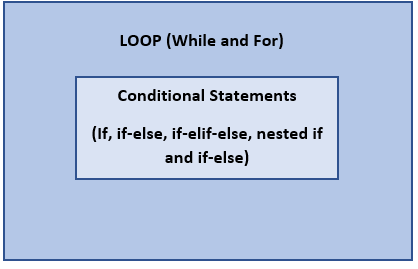Master LLMs with our FREE course in collaboration with Activeloop & Intel Disruptor Initiative. Join now!

## PublicationProgramming

# Understanding Python: Part 4

Last Updated on October 1, 2020 by Editorial Team

#### Conditional statements and loops in python programming with 60+ practice exercises.

After a very detailed study on data structures in Python, let us learn the conditional statements and loops which allows us to do a certain task over and over again(loops) for conditions governed by the conditional statements. We will be seeing each with a few examples to understand how the flow of logic, conditions, iterations are implemented.

NOTE: At the end of this article, I have provided a link to my GitHub repository where you can find more than 60 practice questions and solutions in addition to the examples shown here.

Conditional Statements

if statement:

The if statement decides whether given statements need to be executed or not. It checks for a given condition, if the condition is true, then the code present inside the if block will be executed.

The following figure shows the syntax for “if condition”. If the result of the boolean expression is true, then the code in the if block will be executed.

Example:

We are checking if the string in my_string is a member of the list of strings present in my_list. If yes, then concatenate my_string with “Tutorial”.

if-else statement:

The if-else statement evaluates the test expression and will execute the body of ‘if’ only when the test condition is True.‘If’ decides whether certain statements need to be executed or not. If the condition is false, the body of ‘else’ is executed.

Example:

In the above example, we are checking whether “a”(assigned with 3) and “b”(assigned with 3) are equal. If yes, it prints “A & B are Equal” and if not, it prints “A & B are not Equal”. Since a and b are equal here, the output is “A & B are Equal”.

if-elif-else statement:

The elif is short for else-if. It checks for multiple expressions.
If the condition for ‘if’ is False, it checks the condition for the next elif statement. If all the conditions are False, the else statement is executed.
The if statement can have only one else block but, it can have multiple elif blocks.

Example:

Find the type of the given variable var=1+3j

Here, we have given the different types of data in the multiple elif statements after starting with a condition in the “if”. If the type of the variable var does not belong to any of the types mentioned then the statement which is in the else will get executed.

Nested if and if-else statements:

The nested statements are used to execute different pieces of code when we have more than two options to handle. Nested statements mean that an if statement or if-else statement is present inside another if or if-else block.

Here, we are trying to determine a number given by the user is zero, positive, or negative. Appropriate messages will be printed at the different blocks of the if-else code.

Loops

For loop:

The ‘for’ loop in python is used to execute a block of statements or code several fixed numbers of times by the user. It is used to iterate over a sequence (list, tuple, string) or other iterable objects.

Example:

Find the sum of all the elements in the list “num_list”?

In the above example, we have created a “for” that runs for all the elements in the list num_list and while it runs it adds each element to a variable “total” which holds the sum of all the elements in the list.

While Loop:

While loop executes the same looping procedure by checking a given condition until the condition is false. The condition is given before the loop and is checked before each execution of the loop.

The above figure is a very simple demonstration of while where the loop control variable is initially set to 0 and the loop runs for as long as the expression x<5 holds “True”. For every print of x, the loop control variable x is increased by 1, and the next iteration follows the same process.

break statement:

The break statement is used to stop the loop when a particular condition is true. It is generally used inside a loop along with an if statement so that when a particular condition returns true, the break statement terminates the loop.

In the above example, the task is to print all the elements in the list and exit the loop when the element 838 is encountered.

continue statement:

Continue is also a loop control statement similar to the break statement. But unlike the break statement, it executes the next iteration of the loop instead of terminating the loop. In other words, it skips the iteration for which the condition is given and continues with the next iteration of the loop.

Here in the below example, we are printing all the letters in the word “Python” with a condition that when the iteration element reaches “y”, skip that iteration and continue with the next element. Hence, “y” is excluded in the output.

This completes the study on the most important conditional statements and loops which are very important to have the repeated tasks done. I will be bringing more on the other items in python programming in my upcoming blogs. Till then, Happy reading.!

For more practice, please check out my “Python_Practise” repository in my GitHub wherein there are 8 sets of practice questions which should help you to understand the conditional statements, loops with the various data structures of python. Here is the link,

SuhasVS95 – OverviewUnderstanding Python: Part 4 was originally published in Towards AI — Multidisciplinary Science Journal on Medium, where people are continuing the conversation by highlighting and responding to this story.

Published via Towards AI